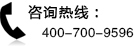### 2014年12月CFA考试大纲：SS 4 Economics: Microeconomic Analysis

201412CFA考试悄然而至，北京国际金融培训中心给大家带来总共18期的《201412CFA考试大纲》，提前帮助大家对CFA考试有一个充分的认识。

201412CFA考试大纲：SS 4 Economics: Microeconomic Analysis

Economics: Microeconomic Analysis

This study session focuses on the microeconomic principles used to describe the marketplace behavior of consumers and firms. The first reading explains the concepts and tools of demand and supply analysis-the study of how buyers and sellers interact to determine transaction prices and quantities. The second reading covers the theory of the consumer, which addresses the demand for goods and services by individuals who make decisions to maximize the satisfaction they receive from present and future consumption. The third reading deals with the theory of the firm, focusing on the sup?ply of goods and services by profit-maximizing firms. That reading provides the basis for understanding the cost side of firms profit equation. The final reading completes the picture by addressing revenue and explains the types of markets in which firms sell output. Overall, the study session provides the economic tools for understanding how product and resource markets function and the competitive characteristics of different industries.

Reading 13 Demand and Supply Analysis: Introduction by Richard V. Eastin and Gary L. Arbogast, CFA

Reading 14 Demand and Supply Analysis: Consumer Demand by Richard V. Eastin and Gary L. Arbogast, CFA

Reading 15 Demand and Supply Analysis: The Firm by Gary L. Arbogast, CFA, and Richard V. Eastin

Reading 16 The Firm and Market Structures by Richard G. Fritz and Michele Gambera, CFA

LEARNING OUTCOMES

READING 13. DEMAND AND SUPPLY ANALYSIS: INTRODUCTION

The candidate should be able to:

a distinguish among types of markets;

b explain the principles of demand and supply;

c describe causes of shifts in and movements along demand and supply curves;

d describe the process of aggregating demand and supply curves;

e describe the concept of equilibrium (partial and general), and mechanisms by which markets achieve equilibrium;

f distinguish between stable and unstable equilibria, including price bubbles, and identify instances of such equilibria;

g calculate and interpret individual and aggregate demand, and inverse demand and supply functions, and interpret individual and aggregate demand and sup?ply curves;

h calculate and interpret the amount of excess demand or excess supply associ?ated with a non-equilibrium price;

i describe types of auctions and calculate the winning price(s) of an auction;

j calculate and interpret consumer surplus, producer surplus, and total surplus;

k describe how government regulation and intervention affect demand and supply;

l forecast the effect of the introduction and the removal of a market interference (e.g., a price floor or ceiling) on price and quantity;

m calculate and interpret price, income, and cross-price elasticities of demand and describe factors that affect each measure.

READING 14. DEMAND AND SUPPLY ANALYSIS: CONSUMER DEMAND

The candidate should be able to:

a describe consumer choice theory and utility theory;

b describe the use of indifference curves, opportunity sets, and budget constraints in decision making;

c calculate and interpret a budget constraint;

d determine a consumers equilibrium bundle of goods based on utility analysis;

e compare substitution and income effects;

f distinguish between normal goods and inferior goods, and explain Giffen goods and Veblen goods in this context.

READING 15. DEMAND AND SUPPLY ANALYSIS: THE FIRM

The candidate should be able to:

a calculate, interpret, and compare accounting profit, economic profit, normal profit, and economic rent;

b calculate and interpret and compare total, average, and marginal revenue;

c describe a firms factors of production;

d calculate and interpret total, average, marginal, fixed, and variable costs;

e determine and describe breakeven and shutdown points of production;

f describe approaches to determining the profit-maximizing level of output;3 Study Session 4

g describe how economies of scale and diseconomies of scale affect costs;

h distinguish between short-run and long-run profit maximization;

i distinguish among decreasing-cost, constant-cost, and increasing-cost indus?tries and describe the long-run supply of each;

j calculate and interpret total, marginal, and average product of labor;

k describe the phenomenon of diminishing marginal returns and calculate and interpret the profit-maximizing utilization level of an input;

l determine the optimal combination of resources that minimizes cost.

READING 16. THE FIRM AND MARKET STRUCTURES

The candidate should be able to:

a describe characteristics of perfect competition, monopolistic competition, oli?gopoly, and pure monopoly;

b explain relationships between price, marginal revenue, marginal cost, economic profit, and the elasticity of demand under each market structure;

c describe a firms supply function under each market structure;

d describe and determine the optimal price and output for firms under each mar?ket structure;

e explain factors affecting long-run equilibrium under each market structure;

f describe pricing strategy under each market structure;

g describe the use and limitations of concentration measures in identifying mar?ket structure;

h identify the type of market structure within which a firm operates.

•北京CFA微信

•金程CFA微博